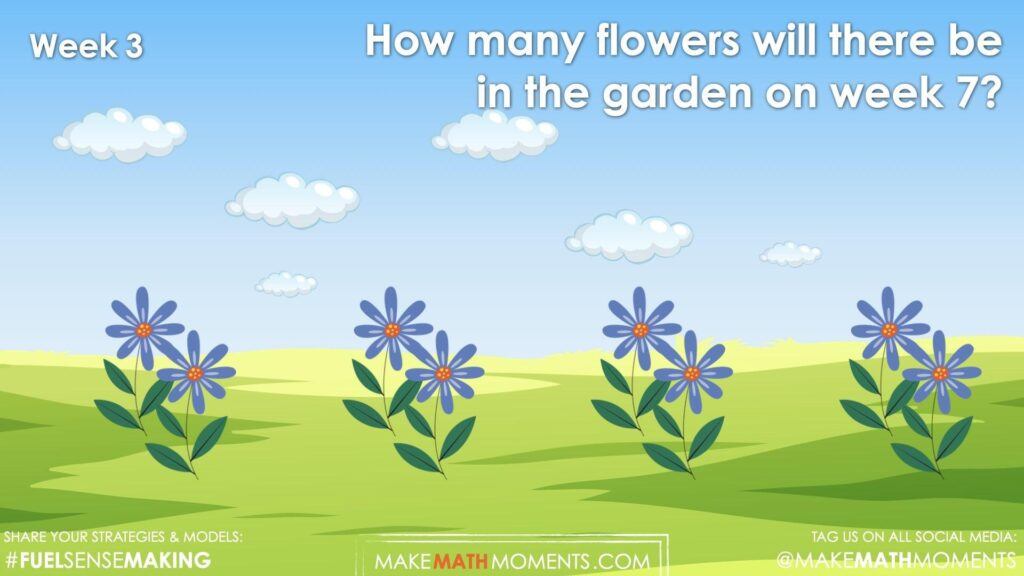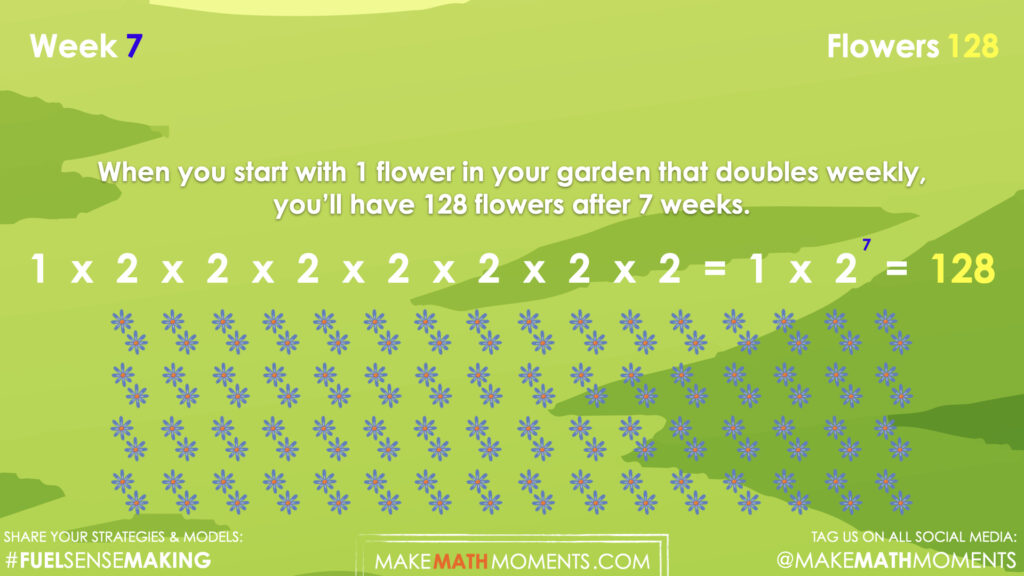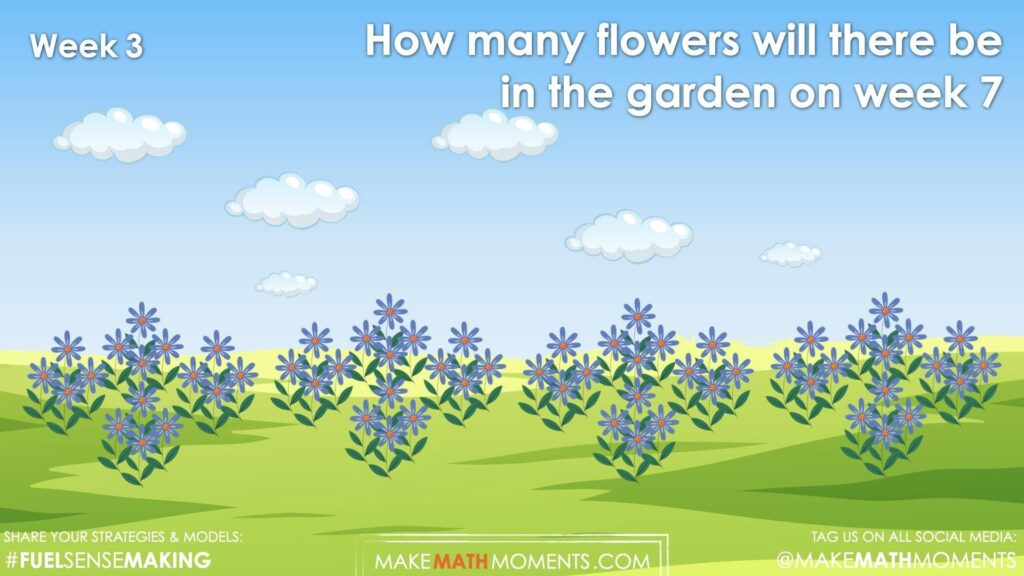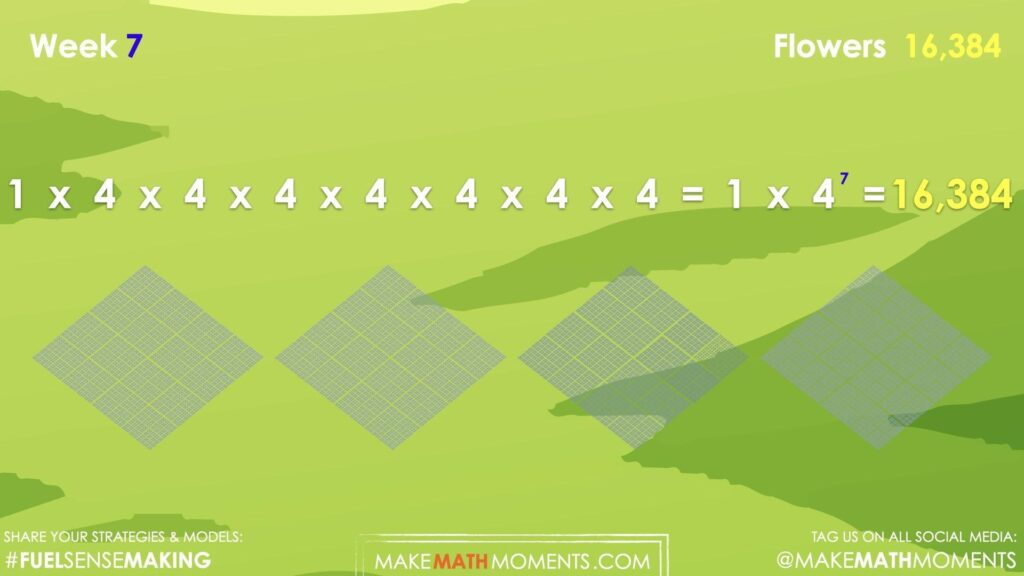## INVASIVE SPECIES [DAY 2]

### EXPONENTIAL GROWTH & DECAY

Through the context of invasive species growth and the use of patterning, students will analyze the relationship between the size and unit of an exponent and the value of a power.

## Intentionality & Unit Overview### Access each lesson from this unit using the navigation links below

Students will extend patterns with exponential growth, make near and far predictions about the pattern, describe the pattern in words, and determine the general term.

## Intentionality…

The purpose of the Day 2 activities is to reinforce key concepts from Day 1. Students will engage in a math talk, solving equations with exponents, and will have an opportunity to complete independent purposeful practice. The math talk and purposeful practice serve to develop a deeper understanding of the following big ideas:

• Patterns can be extended because they are repetitive by nature.
• Pattern rules are generalizations about a pattern, and they can be described in words.
• One common use of patterns is predicting future events.
• A pattern can be extended to make a prediction.
• For far predictions, calculations are required for efficiency.
• Exponential growth is a pattern of data that shows greater increases with passing time,
• An expression that represents repeated multiplication of the same factor is called a power.
• The base number is defined as a number which is multiplied by itself.
• The exponent represents the number of times the base number is multiplied.
• When a pattern is growing exponentially, the variable representing time is the exponent.
• Graphical representations of exponential growth appear as a curved line.

## Math Talk

The following visual number talk is a set of visual patterns that you will share with students one at a time. In order to build on the context from day 1, we will be using the context of weeds (or flowers) growing in a garden. Ask students to describe the change. Collaboratively make a generalization about the relationship between the independent variable, x, and the dependent variable, y, in order to create an exponential general term, written as an expression or as an equation, depending on which you prefer to emerge. Use the exponential expression or equation to determine the value of the 7th term for each exponential relationships.

## Visual Math Talk Prompt #1

Show students the following visual math talk prompt and be prepared to pause the video where indicated:

Students will be prompted with:

How many flowers will there be in the garden by week 7?Students will likely recognize that beginning in week 0, the garden began with 1 flower, then doubled each week afterwards.

By continuing this pattern, students will continue doubling as each week passes until reaching 128 flowers in week 7.Be sure to highlight that since we are doubling each week, we can simply take our original number of flowers (1) and multiply by 2 a total of 7 times – once for each of the 7 passing weeks.

We can write this as:

1 x 2 x 2 x 2 x 2 x 2 x 2 x 2
= 1 x $$2^7$$
= 128

Therefore, you should expect 128 flowers by week 7.

## Visual Math Talk Prompt #2

Show students the following visual math talk prompt and be prepared to pause the video where indicated:

Students will be prompted with:

How many flowers will there be in the garden by week 7?In this visual number talk prompt, the garden once again begins with a single flower with the number of flowers quadrupling each week.Be sure to highlight that since we are quadrupling the number of flowers each week, we can simply take our original number of flowers (1) and multiply by 4 a total of 7 times – once for each of the 7 passing weeks.

We can write this as:
1 x 4 x 4 x 4 x 4 x 4 x 4 x 4
= 1 x $$4^7$$
= 16,384

Therefore, you should expect 16,384 flowers by week 7.

## Visual Math Talk Prompt #3

Login/Join to access the entire Teacher Guide, downloadable slide decks and printable handouts for this lesson and all problem based units.

## Purposeful Practice

Students will have an opportunity to independently complete practice questions related to patterns with exponential growth.

As students are working, you might consider using this opportunity to pose purposeful questions and document student thinking for the purpose of formative assessment.

Pay close attention to the strategies that students are using.

Are students:

• Counting?
• Skip counting?
• Modelling?
• Using the relationship between x and y?
• Using the relationship between the value of each term?
• Using partial products?

While students are working independently, you might also consider strategically pulling students individually or in small groups to offer guided instruction in order to move them along their developmental continuum.

## Questions: Patterns with exponential growth

Question #1:

Login/Join to access the entire Teacher Guide, downloadable slide decks and printable handouts for this lesson and all problem based units.

Question #2:

Login/Join to access the entire Teacher Guide, downloadable slide decks and printable handouts for this lesson and all problem based units.

Question #3:

Login/Join to access the entire Teacher Guide, downloadable slide decks and printable handouts for this lesson and all problem based units.

Question #4:

Login/Join to access the entire Teacher Guide, downloadable slide decks and printable handouts for this lesson and all problem based units.

Question #5:

Login/Join to access the entire Teacher Guide, downloadable slide decks and printable handouts for this lesson and all problem based units.

Login/Join to access the entire Teacher Guide, downloadable slide decks and printable handouts for this lesson and all problem based units.

## Educator Discussion Area

Login/Join to access the entire Teacher Guide, downloadable slide decks and printable handouts for this lesson and all problem based units.

## Explore Our 60+ Problem Based Units

This Make Math Moments Lesson was designed to spark curiosity for a multi-day unit of study with built in purposeful practice, number talks and extensions to elicit and emerge strategies and mathematical models.

Dig into our other units of study and view by concept continuum, grade or topic!